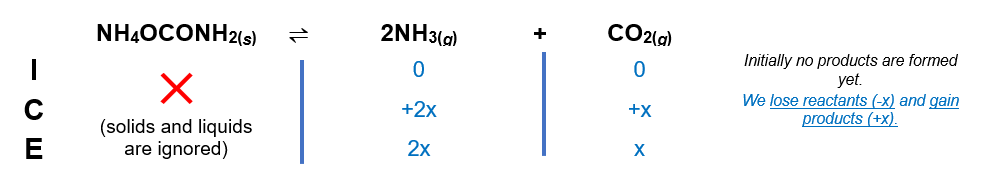# Problem: At 25ºC, Kp = 2.9 x 10 -3 for the reactionNH4OCONH2(s) ⇌ 2NH3(g) + CO2(g)In an experiment carried out at 25ºC, a certain amount of NH4OCONH2 is placed in an evacuated rigid container and allowed to come to equilibrium. Calculate the total pressure in the container at equilibrium.

###### FREE Expert Solution

We are asked to calculate the total pressure in the container at equilibrium.

Step 1. Set up ICE chartStep 2. Calculate x

x = 0.090 atm

95% (289 ratings)###### Problem Details

At 25ºC, Kp = 2.9 x 10 -3 for the reaction

NH4OCONH2(s) ⇌ 2NH3(g) + CO2(g)

In an experiment carried out at 25ºC, a certain amount of NH4OCONH2 is placed in an evacuated rigid container and allowed to come to equilibrium. Calculate the total pressure in the container at equilibrium.